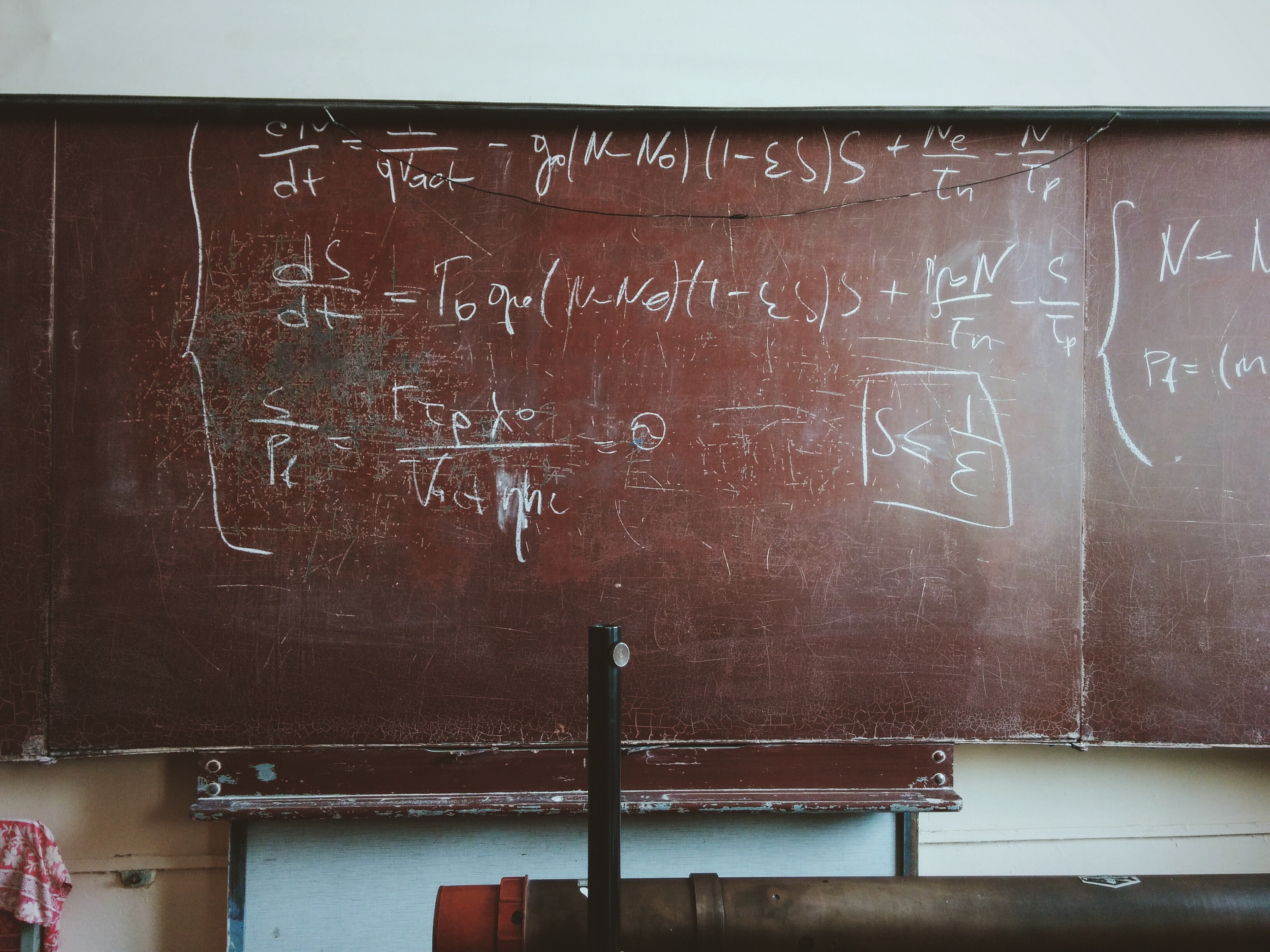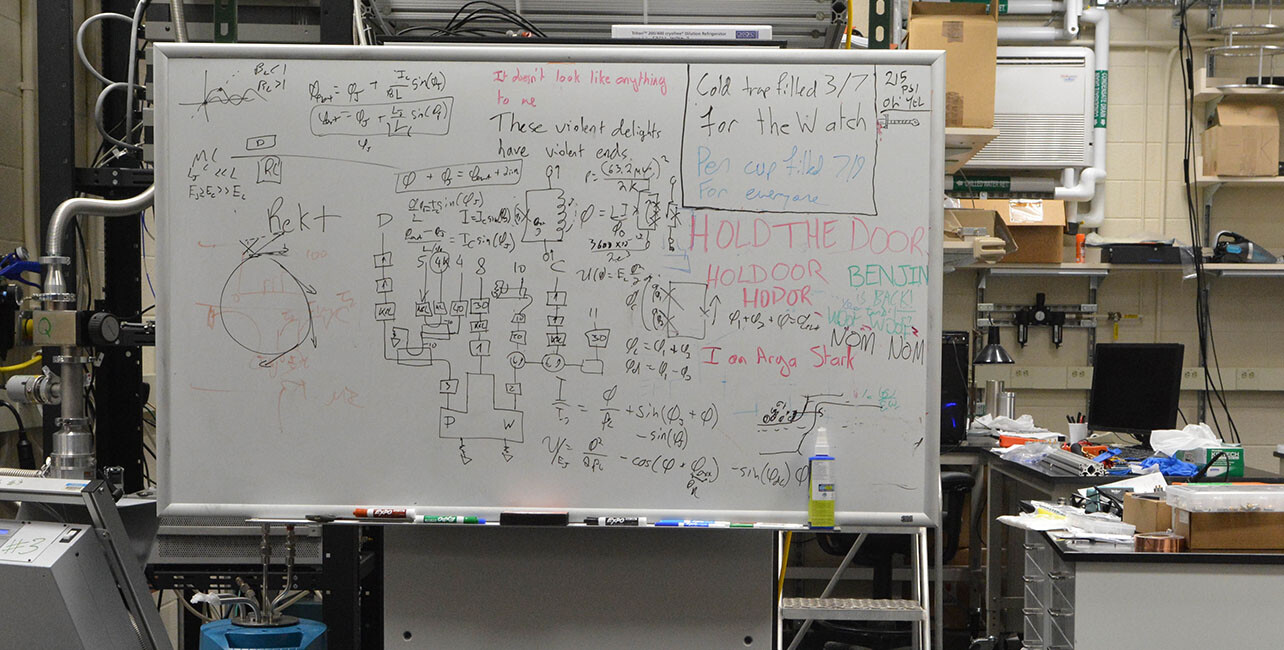Introduction

# The Maths Behind Quantum Computing###### The Maths Behind Quantum Computing

We get it, quantum computing is scary. The combination of quantum mechanics, theories, mathematics, and computer science makes no small hill to climb, and plenty of students have felt discouraged (to put it mildly) at the daunting task.

The keywords “quantum computing for dummies” generate an estimated 480 searches per month, a three-fold increase from the search volume for “quantum computing tutorial”. Of course, this is not meant to point fingers at any self-deprecating individuals out there, but it does illustrate quite well a common dilemma: how does the average person start learning quantum computing?

The answer can be broken down into three primary components: physics, programming, and mathematics. For the moment, let us focus on the mathematics behind quantum computing.

Contrary to popular belief, the mathematics to get started in QC is nowhere near as insane as the chalkboards full of calculational jargon will have you think. Rather, it consists of brief dives into linear algebra and probability.While this whiteboard, with all of its calculations and (admittedly quite) random thoughts, seems daunting, getting started in quantum computing does not require such jargon. (Image courtesy of Yale News)

Of course, it is worth noting that the precise topics to cover may depend upon your chosen profession. Whether you are leaning towards the experimental or theoretical approach will influence the exact depth to which you may be requested to explore.

### Math for Qubits: Linear Algebra

When first approaching any field of study, one will inevitably come across the need to be able to communicate with others. More specifically, one will need to understand the general notation and procedures underlying the primary representations of components within the field of quantum computing. In simpler terms, we are looking for a shared way of representing qubits and their operations.

From a physics perspective, qubits are operated through the manipulation of their spins (vaguely referred to as “intrinsic angular momentum” on an average Internet search, with momentum being a vector quantity) and the direction of the measurement of their spins. Note the keyword here: direction

Enter linear algebra, stage left. In a typical calculus classroom setting, the question of real life applications of linear algebra outside of Newtonian physics is rarely broached. While calculus is not required, the concepts underlying vector mathematics (linear algebra) are useful in describing the spins of particles -- often referred to as the spin model -- and, by extension, qubits.

Regarding the use of matrices and vectors relative to linear algebra, beginning with the fundamentals never fails. For instance, one might suggest knowing how to conduct matrix operations (such as adding and multiplying matrices). This will come in handy in solving problems involving, for example, the probability of measuring the spin of a qubit in some given direction given measurements in another direction.

#### … Differential Equations?

On the question of calculus and (by extension) differential equations, we again come across the differences in emphasis on various components of mathematics. Chad Orzel, an associate professor at Union College, wrote in a Forbes article of the relative importance of solving the harmonic oscillator, especially for experimental physicists.

The quantum harmonic oscillator helps to describe the energy/movement of particles and is often described using an analogy to classical harmonic oscillators (think: mass on a spring). From a distance, it allows for the analytical solving of the Schrodinger equation without the employment of, say, a supercomputer. Knowledge of differential equations is certainly useful in this case.

However, it is worth noting that this particular component is not required to understand the most basic mathematical operations regarding quantum computers, but is useful in grasping the physics underlying their use. In the case you are interested in the quantum physics portion of QC, consider looking into such an area.

### Probability in Quantum Computing

Albert Einstein once infamously quipped “God does not play dice” in response to the assertion that the properties of particles are based upon measurements of certainty, rather than absolute knowledge. The question of certainty -- or, rather, uncertainty -- is a fundamental component of quantum computing, as it plays an integral role in the extraction of information from qubits.

The probability of getting a certain measurement for spin, for one, can be described using probability. Thus, an understanding of probability theory as it relates to quantum mechanics is rather useful.

The concept is illustrated with Heisenberg’s Uncertainty Principle, or, rather, just “The Uncertainty Principle”. The principle was first proposed by Werner Heisenberg in early 20th century Germany and described how the more we know about a particle’s position, the less we know about its momentum.

It rather nicely paints a picture of the difficulty of attempting to pin down precise characteristics of miniscule particles (and, naturally, gives a slight glimpse into the source of Einstein’s frustration).

In general, if you’re worried about getting started in quantum computing, take a small step into linear algebra and probability. There is no telling where you might go with these skills, physics or computer science related or not, but, who knows? You may just crack the code for a QC-related problem you never thought you would be able to solve -- all it takes is a step in the right direction.